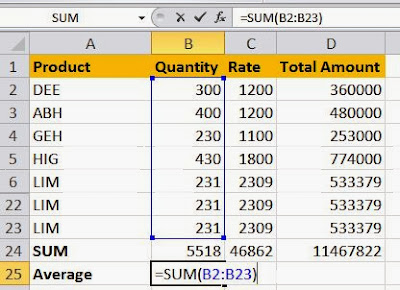## 15 Dec 2013

### Copy the exact formula without shifting range

So you have just entered the SUM formula on 24th row on Excel, and wish to put an AVERAGE formula just below it. If you copy the formula for SUM (fig 1) which is just above it, the range will change automatically (fig 2.)

We will use a simple shortcut to copy the formula ditto (as-is). The quotation mark key on your keyboard operates sort of like a ditto mark shortcut!Fig.1: SUM functionFig 2: Change in range when formula is copied
Simply press (Ctrl + ') key in the 25th row, where you want the formula to be copied. You will see that the formula has been copied without any shift in range.Fig 3: Using Ctrl+'

But our main purpose is not completed yet, so press F2 to change the mode from Enter to Edit.
Go to the beginning of the formula and replace the SUM with AVERAGE and press Enter.

## Disqus

comments powered by Disqus